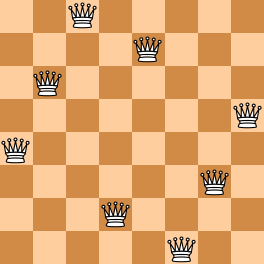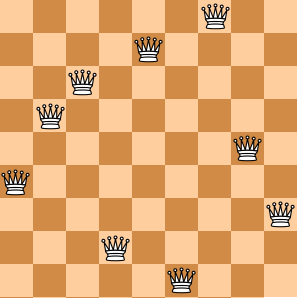# PAT-A 真题 – 1128 N Queens Puzzle

The "eight queens puzzle" is the problem of placing eight chess queens on an  chessboard so that no two queens threaten each other. Thus, a solution requires that no two queens share the same row, column, or diagonal. The eight queens puzzle is an example of the more general  queens problem of placing  non-attacking queens on an  chessboard. (From Wikipedia - "Eight queens puzzle".)

Here you are NOT asked to solve the puzzles. Instead, you are supposed to judge whether or not a given configuration of the chessboard is a solution. To simplify the representation of a chessboard, let us assume that no two queens will be placed in the same column. Then a configuration can be represented by a simple integer sequence , where  is the row number of the queen in the -th column. For example, Figure 1 can be represented by (4, 6, 8, 2, 7, 1, 3, 5) and it is indeed a solution to the 8 queens puzzle; while Figure 2 can be represented by (4, 6, 7, 2, 8, 1, 9, 5, 3) and is NOT a 9 queens' solution.Figure 1 Figure 2

### Input Specification:

Each input file contains several test cases. The first line gives an integer  (). Then  lines follow, each gives a configuration in the format "   ... ", where  and it is guaranteed that  for all . The numbers are separated by spaces.

### Output Specification:

For each configuration, if it is a solution to the  queens problem, print YES in a line; or NO if not.

### Sample Input:

4
8 4 6 8 2 7 1 3 5
9 4 6 7 2 8 1 9 5 3
6 1 5 2 6 4 3
5 1 3 5 2 4

### Sample Output:

YES
NO
NO
YES

#include <iostream>
#include <bits/stdc++.h>
using namespace std;

int main(){
int cnt;
cin >> cnt;
for(int i = 0; i < cnt; i++){
int N;
cin >> N;
//定义存放行、列、斜（下标为行号+列号）的容器
vector<bool>   row(N + 1, false),
col(N + 1, false),
dig(2*(N+1),false);
bool flag = false;  //false=>YES, true=>NO
for(int j = 0; j < N; j++){
int n;
cin >> n;
if(row[n] || col[j] || dig[n + j] || flag){
flag = true;
continue;
}
row[n] = col[j] = dig[n + j] = true;
}
if(flag) cout << "NO" << endl;
else cout << "YES" << endl;
}
return 0;
}